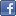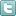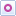EE Times-India > T&M

T&M# Using Python to automate measurements

Posted: 07 Apr 2016Print Version

Keywords:software  LabVIEW  Python  GUI  data-acquisition system

In line 17 we append the dictionary to the results dataframe. Note that results doesn't need to be initialized as well; every time a new line is appended, any new field will be added to the dataframe.

Line 18 is optional, but it can be useful to print the present voltage and current on the terminal, in particular for long measurements, as a way to make sure the application is still running and to know how far along it is.

In lines 19 to 20 the load is turned off and the data is saved on disc. For the latter, each dataframe object has a handy embedded method to save the data on a CSV file.

The power of dataframes
To explore the power of using Python and Pandas dataframes, just add this code between line 16 and line 17.

temp['Vout_id'] = 1.0—2.5e-3*temp['Iout'] # A
temp['Vout_err'] = temp['Vout_id']—temp['Vout'] # B
temp['Pass'] = 'Yes' # C
if (abs(temp['Vout_err']) > temp['Vout_id']*0.001): # D
temp['Pass'] = 'No' # E

Lines A and B generate two new fields of the dataframe. Vout_id contains the ideal DC setpoint value of the output voltage given the measured current and the ideal zero-current setpoint (1 V) and loadline. Vout_err is the absolute error between the ideal and measured voltage.

Lines D and E add the Pass field to the dataframe. The content of the field is a string indicating whether a hypothetic specification of ±0.1% on the output-voltage accuracy is met or not. In figure 3, you can see how the saved CSV file looks in Excel. It's marvelous: numeric data and text are in the same table, and even column headers are automatically generated from the names of the dataframe fields.Figure 3: The Python script can save data in CSV format, which easily opens in Excel.

Data analysis and plotting with Pyplot
The snippet of code described in the previous section allowed us to determine whether the output voltage was within the "tolerance band" around its ideal value. Another interesting piece of information we might want to get out of this experiment is the exact value of the loadline, which is the slope of the VOUT-vs-IOUT curve. If you don't remember how to do a linear fit of the acquired data, don't worry, for Python has a function for it, too. Just insert this code at the end of script:

from scipy.stats import linregress # A
loadline = linregress(results['Iout'], results['Vout']) # B
print "The intercept point is %.3f V" % loadline # D

Line A imports a single method from Scipy's Stats module. In line B, we feed the imported linregress method with the X and Y coordinates of the points we are trying to fit. Finally, we print the result on the terminal in lines C and D. Linregress returns several results organised in an array, with the slope saved at index 0 and the intercept point at index 1. Other information available are the correlation coefficient and the standard error of the estimate.

With such a small dataset (20 points) it is possible to use Excel to generate plots. A three-line example show how that can be done in Python: just add them at the end of the script described previously (the ro parameter of the plot method indicates that I wanted to use red circle markers):

import matplotlib.pyplot as plt # A
plt.plot(results['Iout'],results['Vout'], 'ro') # B
plt.show() # C

Pyplot is a module of the Python's Matplotlib library that contains plenty of methods to plot graphs. Better still, the methods have been designed to be almost identical to MATLAB's. You can see the results of these three lines of code in figure 4. The window and the graphics are automatically generated by Pyplot, and they appear "out of thin air" from the terminal window.Figure 4: Pyplot lets you plot data, which eliminates having to open the data in Excel.

Python is an excellent choice to automate your laboratory setup and avoid tedious hours of measurements because it is simple to use, easy to understand, and extremely flexible and powerful. LabVIEW is, however, still the king of the GUI. In general, I think LabVIEW is better suited for applications requiring a nice graphical interface and that aren't required to execute complex loops or data processing. For instance, I still use LabVIEW to design most of the applications that are customer-facing and so need to be pretty but are rarely complicated. For all the other applications and automation needs, though, Python is now my first choice.

What is Python and why use it?
Python is an interpreted, object-oriented, high-level programming language with dynamic semantic. Since its first release in 1991, Python has grown in popularity and it is now used in a wide range of applications; it is one of the most commonly taught programming languages in major universities and online courses. What makes Python such a great "first" programming language are its simplicity and easy-to-learn syntax and readability (some say "it is written in plain English"), all combined with great versatility and capabilities.

 Related Articles Editor's Choice
Comment on "Using Python to automate measurement..."

Top Ranked Articles

WebinarsVisit Asia Webinars to learn about the latest in technology and get practical design tips.

Search EE Times India
Services

Go to topConnect on FacebookFollow us on TwitterFollow us on Orkut
﻿